# Dino Cube facts for kids

Kids Encyclopedia Facts

The Dino Cube is a cubic twisty puzzle in the style of the Rubik's Cube. It was invented in 1985 by Robert Webb, however it was not mass-produced until ten years later. It has a total of 12 external movable pieces to rearrange, compared to 20 movable pieces on the Rubik's Cube.

## Overview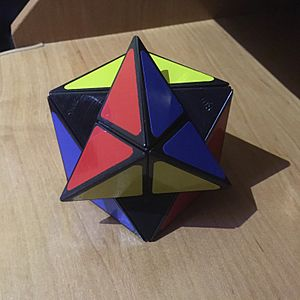The six-colour Dino Cube in the middle of a move, showing how the puzzle can be scrambled. Note the black pieces inside of the puzzle: these are "hidden" corner pieces which form the core of the puzzle.

The Dino Cube is a twisty puzzle in the shape of a cube. It consists of 12 movable pieces, all of which are located on the edges of the cube.

The puzzle can be thought of as twisting around its corners: each move changes the position of three edge pieces adjacent to the same corner, by rotating them around that corner. There are in fact eight more "hidden" pieces inside the puzzle, which are located at the corners and are fixed to the puzzle's core; these pieces only become visible in the middle of a move.

The vast majority of mass-produced Dino Cubes have the standard six-colour scheme, with one colour on each face of the cube in the solved state. This is in common with most other cubic twisty puzzles, including the Rubik's Cube. However, a few versions with other colour schemes also exist, including one with four colours (where each colour is centred around one corner of the cube in the solved state), and one with just two colours (where each colour present on half of the puzzle).

The purpose of the puzzle is to scramble the colours and then restore them to their original configuration, usually of one colour per face.

## Solving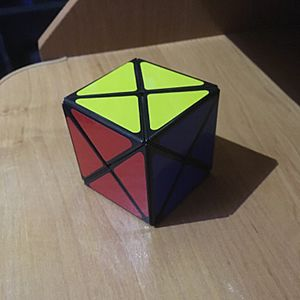The Dino cube in its second solved state, which is a mirror image of the first one.

The Dino Cube is considered to be one of the easiest twisty puzzles to solve. One of the things that make it so easy is the fact that each move only affects three edge pieces at once, which means it is easy to solve one part of the puzzle without disturbing what is already solved. In addition, each edge piece only has one possible orientation, meaning that if a given piece is in the correct position, it will always be orientated the correct way as well. Therefore, the solver never has to worry about changing the orientation of any pieces.

Although not obvious at the first glance, the six-colour Dino Cube actually has two distinct configurations that represent a solved puzzle. The two solutions are mirror images of each other and the only visual difference between them is their colour schemes; for example, one solution has the colours Blue - Yellow - Red going clockwise around one vertex, while in the other these colours go anticlockwise.

Mathematically, the puzzle is identical to Hoberman's BrainTwist, which is a tetrahedral puzzle that can "flip" inside out and reveal another set of four faces. Like the Dino Cube, The BrainTwist has twelve movable pieces, and each move rotates three pieces around one corner. It also likewise has two distinct solutions: one with the same colour on each of the faces and one with the same colour at each of the corners.

## Number of combinations

The Dino Cube has twelve edge pieces. This means naturally there are twelve possible positions for the first given edge, however due to the lack of visible fixed "reference" pieces, all of these positions are rotationally symmetrical to each other. Therefore, the position of the first given edge is not taken into account.

The remaining eleven edge pieces can be permutated in 11! different ways, relative to the first edge piece. Only even permutations of these pieces are possible (i.e. it is impossible to swap one pair of pieces while leaving the rest of the puzzle solved), which divides the limit by 2.

The edge pieces cannot be flipped or misorientated (See Solving), therefore this is also not taken into account.

The total number of possible combinations on the six-colour Dino Cube is therefore equal to: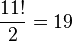$\frac{11!}{2} = 19$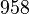$958$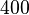$400$

This number is low compared to the number of combinations of the Rubik's Cube (which has over 4.3×1019 combinations) but still larger than many other puzzles in the Rubik's Cube family, notably the Pocket Cube (over 3.6 million combinations) and the Pyraminx (just over 930 thousand combinations, excluding rotations of the trivial tips).

### Optimal solutions

The number of possible of configurations, 19 958 400, is sufficiently small to allow a computer search for optimal solutions. The table below summarises the result of such a search, stating the number p of positions that require n moves to solve the six-colour Dino Cube (p2 for either solution, p1 for one specific solution):

n 0 1 2 3 4 5 6 7 8 9 10 11 Total
p2 2 32 320 2 816 23 414 180 084 1 227 084 6 167 660 10 926 002 1 429 972 1 004 19 958 400
p1 1 16 160 1 408 11 712 90 912 640 192 3 740 838 11 138 597 4 313 963 20 577 24 19 958 400

This table shows that the God's Number of the six-colour Dino Cube is 10 (when solving into either solution) or 11 (when solving into one specific solution).

## Variations

Several shape modifications of the Dino Cube exist. These include the aforementioned BrainTwist, in the shape of a tetrahedron that can flip inside out, the Platypus, whose shape is also based on a tetrahedron, the Redi Cube, a cubic version with shallower cuts, and the Rainbow Cube, in the shape of a cuboctahedron. The latter three puzzles, unlike the Dino Cube, also have their "core" pieces visible.Dino Cube Facts for Kids. Kiddle Encyclopedia.